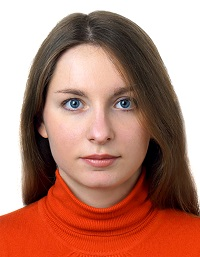## Time step in FlowVision. How to specify

To solve a numerical task, it is necessary to discretize space and time. A time scale allows to find intermediate solutions based on the initial and boundary conditions. Gradually coming through the intermediate solutions, we get the final one, which we can use to achieve the goals: choosing the right paint for the rocket skin, calculating the flow rate of your favorite ketchup, or getting the optimal washing temperature for a cat.

The intervals at which software calculates a solution are commonly referred to as the time step. It can be constant or calculated for each iteration based on a certain criteria. It should be noted that the time step is different for each specific software and task.

How to specify it correctly - we will describe in this article.

### Time step in FlowVision

FlowVision allows to set time step as:

• Constant – differential equations will be solved through the equal time intervals during the simulation;
• CFL number – the Courant-Friedrichs-Levy condition (CFL condition) is used. It controls the time step based on the current simulation characteristics.

#### constant time step

Everything is quite boring about constant time step - the timeline is splitted for the required interval (usually less than 0.1 of the characteristic time) and all you have to do - just wait until either the solution converges or the specified computation time ends.

The most common tasks that are solved with constant time step:

• Flow in a tube. The characteristic time for this task is transit time;
• External flow around the body. Characteristic time - the ratio of the body size to the incoming flow velocity;
• Circulating flow. The characteristic time is the time for which a flow particle makes a revolution in the computational domain.

#### CFL number

The CFL criteria are a little more complicated. The criterion itself is a necessary condition for the stability of an explicit numerical solution of partial differential equations. That is, the time step should be less than a certain value, otherwise the simulation results will be non-physical.

To make it clear - FlowVision itself calculates the time step with which it will be comfortable to make calculations based on the already obtained solution. And the user only scales this value.

The step that FlowVision calculates by itself is called the explicit time step (τexpl). The scaling factor is the CFL number. The final time step (τ) is calculated by the formula: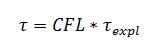If you open Solver tab > Time step, you may see that there are 4 criteria:

• Convective CFL;
• Surface CFL;
• Diffusive CFL;
• Slide CFL.

Each criterion has its' own explicit step, which is calculated by FlowVision:

• Convective explicit step τexpl,conv
• Surface explicit step τexpl,surf
• Diffusive explicit step τexpl,diff
• Slide explicit step τexpl,slide

The limiter for the above steps is

• Gravitational step  τgr .

Maybe, you have a lot of questions already:

### Why are there so many criteria and explicit steps?

FlowVision simulates various processes for which it is necessary to solve different equations: equation of fluid motion, multiphase interaction, heat transfer, mass transfer and many others. One or another criterion for calculating the time step is selected depending on the problem to be solved, .

Let's consider a boat floating along the waves. The following processes are modeled to obtain a solution of such task:

• Water motion;
• Boat movement;
• Free surface motion - phase boundary: water - air.

Diffusion proccesses and sliding surface are not taken into account, that means there is no point in considering these criteria for calculating the final time step for this particular task. The convective and surface criteria are used.

It is necessary to specify surface CFL = 1 for tasks with moving bodies.

Or another example: the problem of particles mass transfer. The processes, that are modelled in this task:

• Phase motion;
• Motion of dispersed particles.

There is still no sliding surface, also there is no moving bodies and free surface movement. Therefore, only convective and diffusion criteria will be used.

If you have a rotation with sliding surfaces it is neccessary to spesify slide CFL = 1

If a physical process that defines the criterion is not considered in your task, then the criterion (CFL number) should be assigned the value 1e + 20. In such a setting, the criterion will not be taken into account.
Let's have a look at each of the criteria and the corresponding explisit time step.

#### convective CFL

Is used in the tasks, where motion is simulated.

General recommendation for choosing a value for this criterion is to perform an investigation on this time step.

All tasks are different and require own specific time step. Therefore, it is necessary to investigate the dependence of the characteristic control value on the time step.
For example, examining the pressure drop in a pipe with a variable velocity at the inlet, it can be noted how the value of the pressure drop is changing at different steps. At a certain value of the time step (t <20), the control value doesn't change - this is the required time step.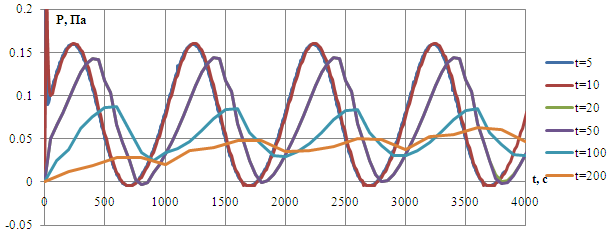The time step is calculated as follows: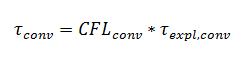Convective CFL scales convective time step, which is calculated by formula: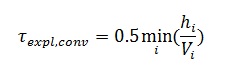To calculate the explicit time step, FlowVision determines the minimum ratio of a cell size to velocity in that cell within the entire subregion. If you know the size of a cell and can estimate a velocity value for a specific task, you can also calculate the step in volume by the formula.

For supersonic flows, it is recommended to use convective CFL 1 ... 10, for subsonic flows it is possible to start with higher values 30…100 CFL.

#### Diffusive CFL

Is used for diffusive time step calculation: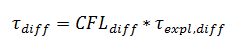It means, the diffusive CFL also scales explicit diffusive time step: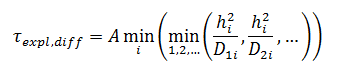where A = 1 is set for implicit scheme and A = 0.25 - for explicit scheme, ensuring its stability.

The explicit diffusive step is calculated as the minimum time during which the velocity perturbation propagates diffusionally in the cell. This step is used for viscous flows simulations, chemical reactions and anisotropy.

It is recommended to set up diffusive CFL from 10 to 1000.

#### surface CFL

It is used in any tasks where the motion of a free surface or moving bodies is simulated. This criterion limits the step so that the body or surface cannot cross more than one cell in one iteration. If the body will cross more than one cell at a time, then the calculation will turn out to be far from physical.

The step size for such tasks is appropriate: surface CFL= 1.

It is calculated as: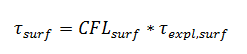where the explicit step is calculated by FlowVision as minimum of the ratio of the size of a cell containing a free surface or a moving body to the velocity modulus at the phase/moving body boundary: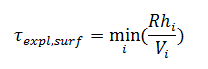R –coefficient that increases the stability of calculation. For a free surface, R = 0.5, and for a moving body, R = 1.

#### Slide CFL

Calculates the time step for a sliding surface: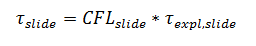Explicit time step is calculated as the minimum ratio of the cell size on a sliding surface to the linear velocity on this sliding surface.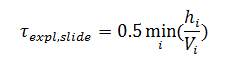It is specified strictly as 1.

If a "frozen rotor" is used - it is not taken into account in time step calculation.

#### Then how IS a time step CALCULATED, ONCE THEre are so many CRITERIA AND explicit STEPS?

The time step itself is calculated on the base of the minimum value of the time steps obtained using all the specified criteria: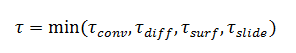In addition to the fact that the minimum value is selected, the step limiters are also taken into account. Time step is compared with the minimum and maximum time steps that are set by user: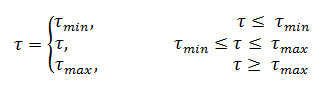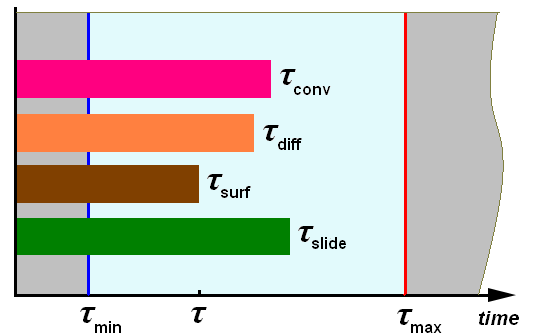#### why do we need gravitational time step? and why doesn't it have a criterion?

The gravitational time step is set for the projects where the gravity vector is specified. The presence of gravity can accelerate the body or slow it down significantly. Accordingly this can increase or decrease the time step. To limit the surface and convective explicit steps from above, the gravitational step is calculated.

It is calculated by formula: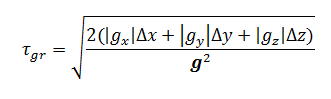Where:

• g - gravity vector;
• gcomponent of gravity vector;
• Δi - minimal cell size.

#### Life hacks

• During the simulation, it is necessary to understand what physics is currently affecting the solution and check the characteristic step for this problem periodically.

• If the time step "settles in" (i.e. decreases sharply) in relation to the expected time step, you need to think about whether everything is set and calculated correctly. The simpliest diagnostics is to display "Internal characteristics" in the monitor window, such as:

• Time step;
• Explicit time step (calculated as an explicit convective step).
• To make it easier to find the area due to which the step settles in, FlowVision can display quantities both in volume and on a plane.

• Is it possible that the time step becomes less than an explicit time step?
Yes, this is possible, since the time step in FlowVision is counted twice per iteration. A time step is calculated on the base of all steps at the beginning of an iteration. After that, the moving body is inserted and the explicit convective step is recalculated. A new time step is not calculated! Therefore, it is quite possible that the new explicit time step will be larger than the time step.